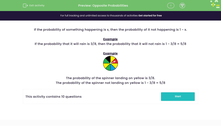# Calculate Opposite Probabilities

In this worksheet, students will calculate the probability of events not happening.Key stage:  KS 3

Curriculum topic:   Probability

Curriculum subtopic:   Understand Probability Outcomes

Popular topics:   Probability worksheets

Difficulty level:#### Worksheet Overview

If the probability of something happening is x, then the probability of it not happening is 1 - x.

Example

If the probability that it will rain is 3/8, then the probability that it will not rain is 1 - 3/8 = 5/8

ExampleThe probability of the spinner landing on yellow is 3/8.

The probability of the spinner not landing on yellow is 1 - 3/8 = 5/8

Let's have a go at some questions now.

If the probability of you getting all the questions correct is 9/10, what's the probability that you don't get them all correct?Could it be 1 - 9/10, which is 1/10?!

### What is EdPlace?

We're your National Curriculum aligned online education content provider helping each child succeed in English, maths and science from year 1 to GCSE. With an EdPlace account you’ll be able to track and measure progress, helping each child achieve their best. We build confidence and attainment by personalising each child’s learning at a level that suits them.

Get started